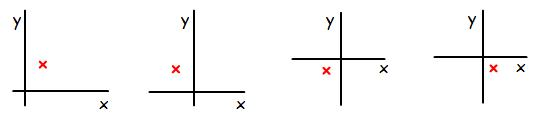#### You may also like### Equation Matcher

Can you match these equations to these graphs?### Curve Fitter

Can you fit a cubic equation to this graph?### Guess the Function

This task depends on learners sharing reasoning, listening to opinions, reflecting and pulling ideas together.

# Real-life Equations

##### Age 16 to 18 Challenge Level:

This is a list of many of the most important equations in science. In each case, we have labelled the two variable quantities $x$ and $y$. The letters $a, b$ stand for constants in each case

Constant motion $\quad\quad\quad\quad\quad a = \frac{x}{y}$

Constant acceleration $\quad\quad\quad x = uy + \frac{1}{2} ay^2$

Beer Lambert Law $\quad\quad\quad\quad a=bxy$

Exponential decay $\quad\quad\quad\quad x=a e^{by}$

Michaelis-Menton $\quad\quad\quad\quad x = \frac{ay}{b+y}$

pH $\quad\quad\quad\quad\quad\quad\quad\quad\quad x = -\log_{10}(y)$

Can you identify the possible meanings of the variables $x$ and $y$ and the constants in each case?Four graphs are shown above, where the two axes intersect at the origin $(0, 0)$.

The red crosses show four measurements. Although we do not know the numerical values (because there are no scales on the graphs), we can see whether the values are positive or negative in each variable. For example, the first measurement is positive in $x$ and positive in $y$; the second measurement is positive in $y$, negative in $x$.

For processes evolving according to each of the equations above, which measurements are possible?